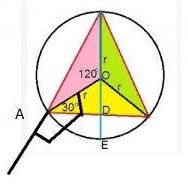# Portion of Altitude of a Triangle Inscribed in a Circle

zak100

## Homework Statement

In the figure Q image2.jpeg (attached), equilateral triangle ABC is inscribed in circle O, whose radius is 4. Altitude BD is extended until it intersects the circle at E. What is the length of DE?

Solution figure is attached. They formed a right angled triangle & calling it 30-60-90. I know the 90 degree angle but how to find the vertices with 30 degrees & 60 degrees angle?

## Homework Equations

Pythagorous thorem:
sqr(a) + sqr(b) = sqr(c)
Sides of a 30-60-90 tirangle are : x, x * sqrt(3), 2 * x (hypotenuse)

## The Attempt at a Solution

The solution image is attached. They connected A with O. It then forms a triangle AOD. AO is 4 because its radius (no problem). Therefore 2 * x = 4, so x = 2, so length of smaller side is 2. But which side is smaller. For this we must know the angles associated with the vertices A & O.

Some body please tell me the angle formed by the vertices A & O, how should we find this?

Zulfi.

Zulfi.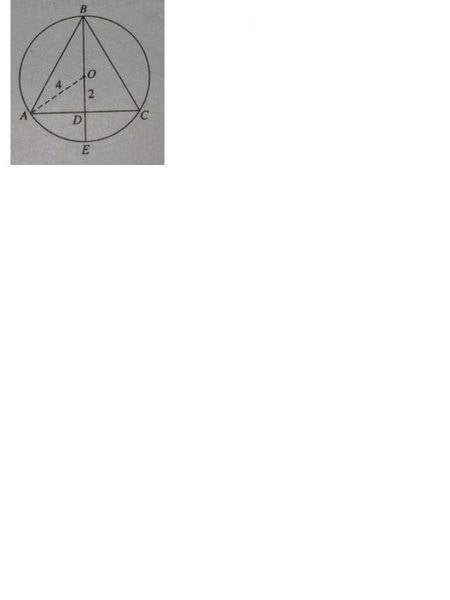.

Staff Emeritus
Homework Helper

## Homework Statement

In the figure Q image2.jpeg (attached), equilateral triangle ABC is inscribed in circle O, whose radius is 4. Altitude BD is extended until it intersects the circle at E. What is the length of DE?

Solution figure is attached. They formed a right angled triangle & calling it 30-60-90. I know the 90 degree angle but how to find the vertices with 30 degrees & 60 degrees angle?

## Homework Equations

Pythagorous thorem:
sqr(a) + sqr(b) = sqr(c)
Sides of a 30-60-90 tirangle are : x, x * sqrt(3), 2 * x (hypotenuse)

## The Attempt at a Solution

The solution image is attached. They connected A with O. It then forms a triangle AOD. AO is 4 because its radius (no problem). Therefore 2 * x = 4, so x = 2, so length of smaller side is 2. But which side is smaller. For this we must know the angles associated with the vertices A & O.

Some body please tell me the angle formed by the vertices A & O, how should we find this?

Zulfi.

Zulfi.View attachment 103217 .
If the hypotenuse of the 30-60-90 triangle is 4, and the altitude is 2, what length is the base? Apply the Pythagorean Theorem here.

You know that the center of the circle is at O. What must be the total length of the line BOE which passes thru O? If you know the length of BOE, then you should be able to find the length DE

zak100
Hi,
No altitude 2 is not given. Sorry I have uploaded the solution part of the figure. The figure related to question is: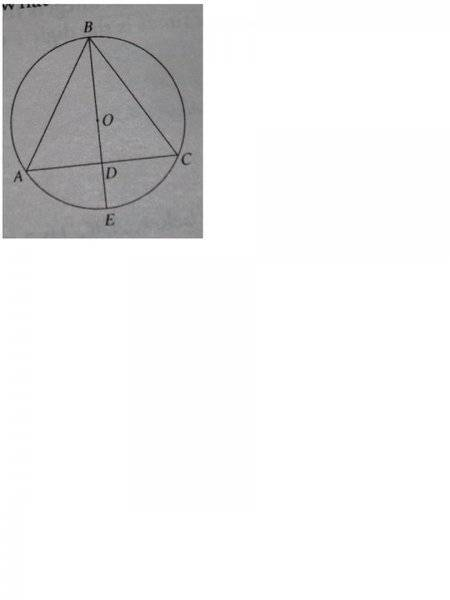< If you know the length of BOE,>
According to the question i dont know the length of DE. Actually, i can find the lengths of other 2 sides of ADB if i know the length of hypotenuse using the formulas. But i dont know whether A is 30 or B is 30. This is what i want to know. How can we know which angle is 30 & which angle is 60. For this we must know which side is larger. The angle opposite to the larger side would be 60. We cant believe on the drawing. So without considering the drawing how can we find out which side is larger?

Zulfi.

Homework Helper
Gold Member
Hi,
No altitude 2 is not given. Sorry I have uploaded the solution part of the figure. The figure related to question is:View attachment 103317
< If you know the length of BOE,>
According to the question i dont know the length of DE. Actually, i can find the lengths of other 2 sides of ADB if i know the length of hypotenuse using the formulas. But i dont know whether A is 30 or B is 30. This is what i want to know. How can we know which angle is 30 & which angle is 60. For this we must know which side is larger. The angle opposite to the larger side would be 60. We cant believe on the drawing. So without considering the drawing how can we find out which side is larger?

Zulfi.

Draw lines AO and OC. This gives you three congruent isosceles triangles AOB, BOC, and COA since they have equal corresponding sides. So their central angles are equal and add to 360 degrees. So each central angle is 120 degrees. Since the triangles are isosceles their smaller angles must all be 30 degrees. Does that help you?

zak100
Hi,
My friend thanks for your guidance. This wont help me at all. Because I have to find DE. If i can find OD, i can subtract BE from BD & this would give me OD. None of these triangles contain the sides DE which is required in the question.

Zulfi.

Homework Helper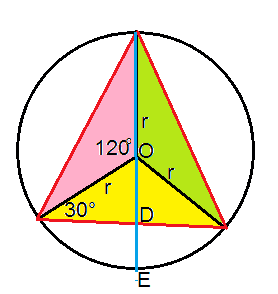The red triangle is equilateral. All the shaded triangles are congruent. Therefore the central angles are 120°. They are isosceles, with sides of length r. The line OE halves the triangle ACO, so OD is perpendicular to AC. What is the length of OD then? OE is one radius of the circle. What is DE?

zak100
Hi,
Thanks for your work. It looks that we now have 30-60-90 triangle. But I have a feeling that 30 degree is not correct. Because its an exterior angle of the triangle and exterior angle is the sum of two opposite interior angles.

Zulfi.

Homework Helper
Hi,
Thanks for your work. It looks that we now have 30-60-90 triangle. But I have a feeling that 30 degree is not correct. Because its an exterior angle of the triangle and exterior angle is the sum of two opposite interior angles.

Zulfi.
Do you see any triangle with exterior angle of the 30° shown?
http://www.mathopenref.com/triangleextangle.html

zak100
Hi,
Thanks for sending me the link. I was wrong. I now understand what is meant by an exterior angle. I also understand why the angle is 120. The angle around O would be 360 & 360 degree divided by 3 is equal to 120 degrees. Line OD halves the triangle so angle AOD in my diagram would be 60 degrees. So larger side would be AD= x * sqrt(3) & x =2 & the length of smaller side OD = x =2 & DE would be 2.

Thanks.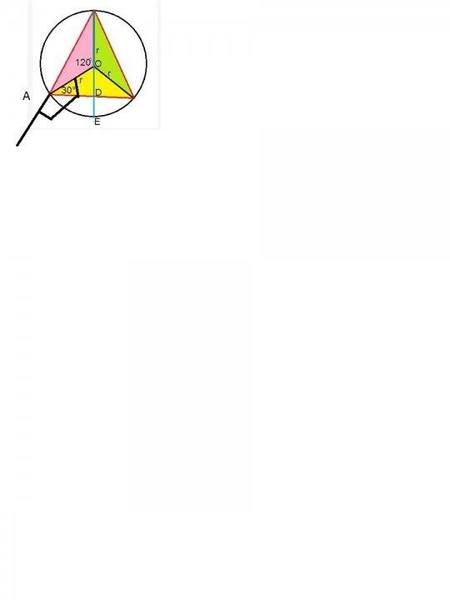Sorry for extra white space in the image. When I load image i get this problem.
Zulfi.

Homework Helper
Hi,
Thanks for sending me the link. I was wrong. I now understand what is meant by an exterior angle. I also understand why the angle is 120. The angle around O would be 360 & 360 degree divided by 3 is equal to 120 degrees. Line OD halves the triangle so angle AOD in my diagram would be 60 degrees. So larger side would be AD= x * sqrt(3) & x =2 & the length of smaller side OD = x =2 & DE would be 2.

Thanks.
View attachment 103422
Sorry for extra white space in the image. When I load image i get this problem.
Zulfi.
Good work!
From the image, select the relevant part. Copy it and paste into your post.